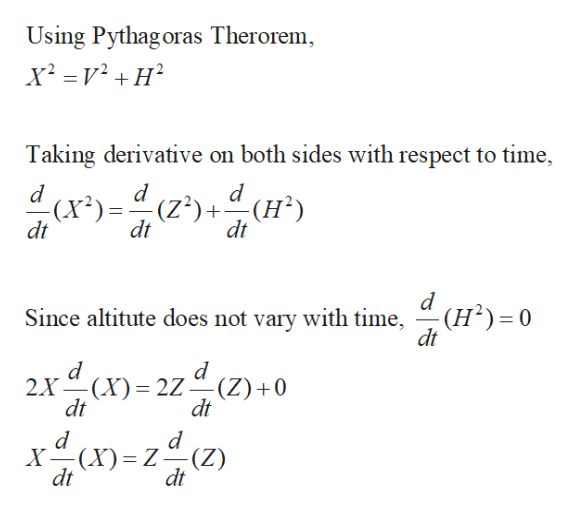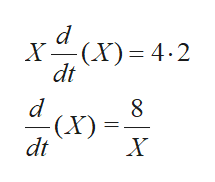A helicopter flies parallel to the ground at an altitude of 1/2 kilometer and at a speed of 2 kilometers per minute. If the helicopter passes directly over the White House, at what rate is the distance between the helicopter and the White House changing 1 minute after the helicopter flies over the White House?1 minute after the helicopter passes over the White House, its distance from the White House is changing at a rate of ______ kilometers per minute.

Question

A helicopter flies parallel to the ground at an altitude of 1/2 kilometer and at a speed of 2 kilometers per minute. If the helicopter passes directly over the White House, at what rate is the distance between the helicopter and the White House changing 1 minute after the helicopter flies over the White House?

1 minute after the helicopter passes over the White House, its distance from the White House is changing at a rate of ______ kilometers per minute.

Step 1

The numerical can be constructed as shown below,
let H be the altitude = 0.5 km

V be the speed =2 km/min

The horizontal distance z travelled at the end of 2 minutes will be 2km/min* 2 min=4 kms

Step 2

Let the distance from the white house be given by the red line denoted by X. It can be expressed in terms of V and H as,help_outlineImage TranscriptioncloseUsing Pythag oras Therorem Taking derivative on both sides with respect to time, d d (x2)(Z2)+(H2 dt dt ) dt Since altitute does not vary with time, (H2) 0 dt 2X(X dt 2Z-- (Z) 0 dt (X)= z(z) X dt Z dt fullscreen
Step 3

Substitute the values an...help_outlineImage TranscriptioncloseX(X 4.2 dt - (X) dt X fullscreen

Want to see the full answer?

See Solution

Want to see this answer and more?

Our solutions are written by experts, many with advanced degrees, and available 24/7

See Solution
Tagged in

Derivative